# dc1.pdf

11 de Apr de 2022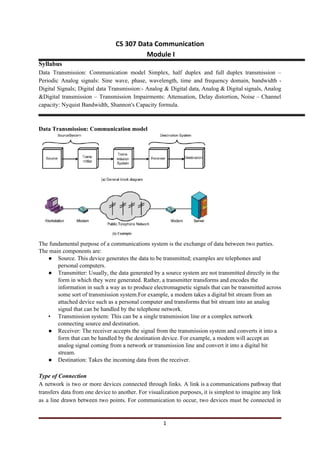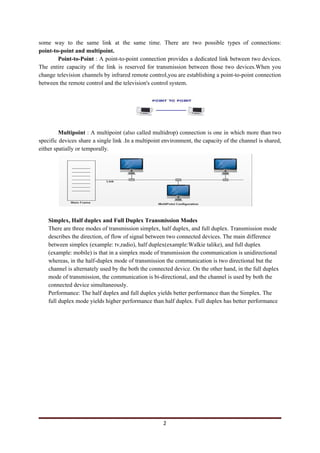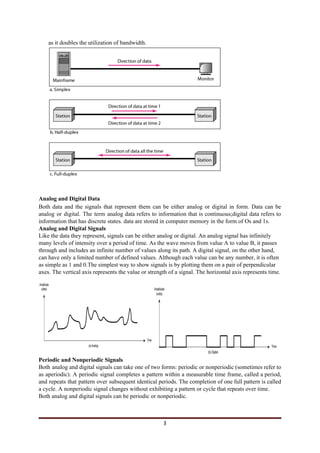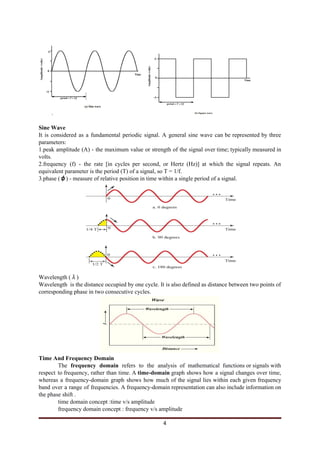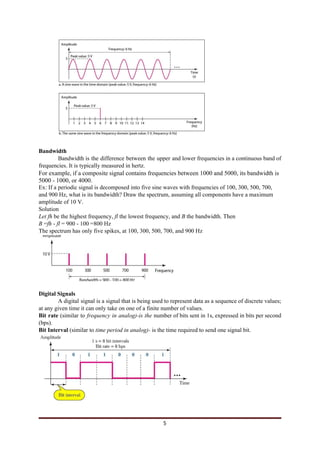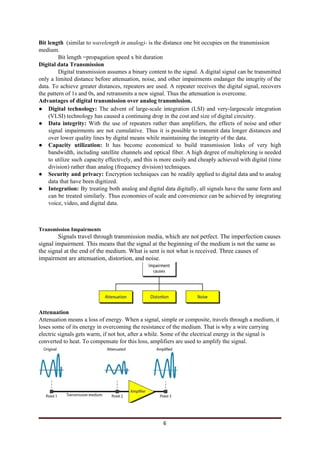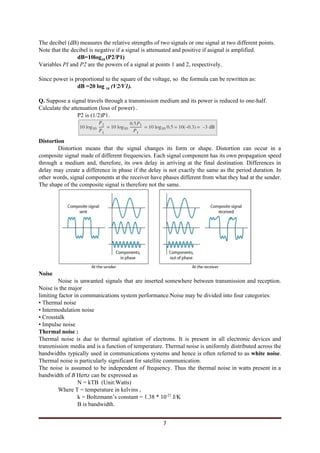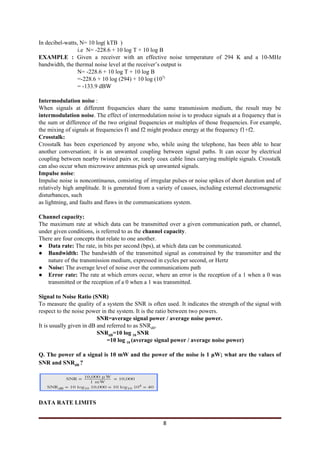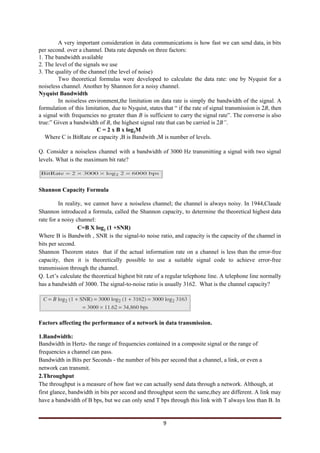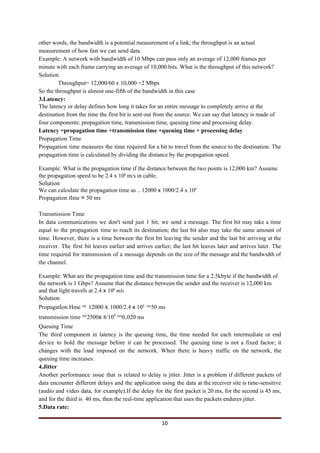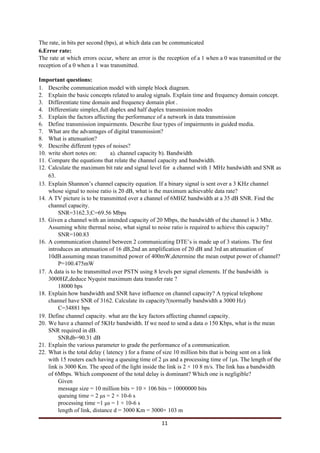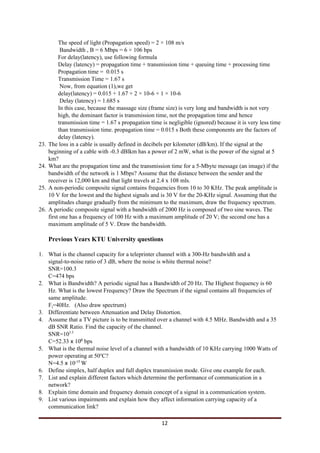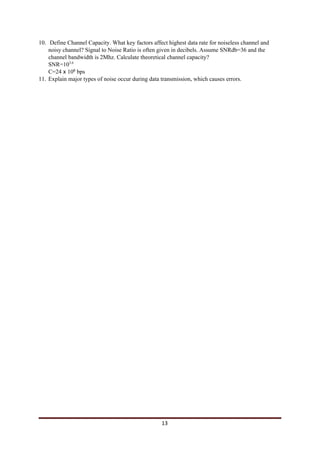Próximo SlideShare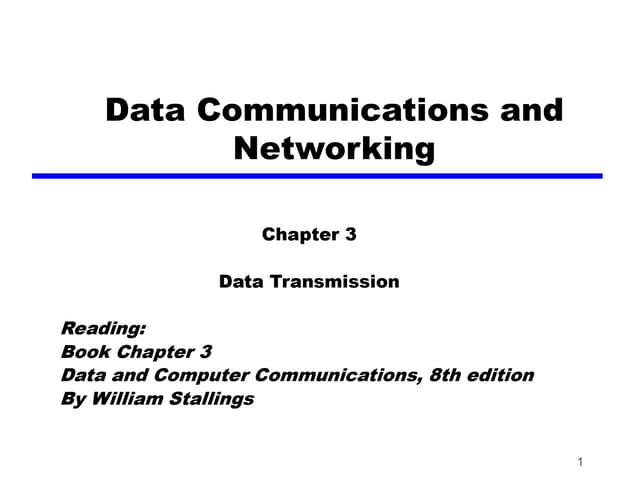Ch03 2
Cargando en ... 3
1 de 13

### dc1.pdf

1. CS 307 Data Communication Module I Syllabus Data Transmission: Communication model Simplex, half duplex and full duplex transmission – Periodic Analog signals: Sine wave, phase, wavelength, time and frequency domain, bandwidth - Digital Signals; Digital data Transmission:- Analog & Digital data, Analog & Digital signals, Analog &Digital transmission – Transmission Impairments: Attenuation, Delay distortion, Noise – Channel capacity: Nyquist Bandwidth, Shannon's Capacity formula. Data Transmission: Communication model The fundamental purpose of a communications system is the exchange of data between two parties. The main components are: ● Source. This device generates the data to be transmitted; examples are telephones and personal computers. ● Transmitter: Usually, the data generated by a source system are not transmitted directly in the form in which they were generated. Rather, a transmitter transforms and encodes the information in such a way as to produce electromagnetic signals that can be transmitted across some sort of transmission system.For example, a modem takes a digital bit stream from an attached device such as a personal computer and transforms that bit stream into an analog signal that can be handled by the telephone network. • Transmission system: This can be a single transmission line or a complex network connecting source and destination. ● Receiver: The receiver accepts the signal from the transmission system and converts it into a form that can be handled by the destination device. For example, a modem will accept an analog signal coming from a network or transmission line and convert it into a digital bit stream. ● Destination: Takes the incoming data from the receiver. Type of Connection A network is two or more devices connected through links. A link is a communications pathway that transfers data from one device to another. For visualization purposes, it is simplest to imagine any link as a line drawn between two points. For communication to occur, two devices must be connected in 1
2. some way to the same link at the same time. There are two possible types of connections: point-to-point and multipoint. Point-to-Point : A point-to-point connection provides a dedicated link between two devices. The entire capacity of the link is reserved for transmission between those two devices.When you change television channels by infrared remote control,you are establishing a point-to-point connection between the remote control and the television's control system. Multipoint : A multipoint (also called multidrop) connection is one in which more than two specific devices share a single link .In a multipoint environment, the capacity of the channel is shared, either spatially or temporally. Simplex, Half duplex and Full Duplex Transmission Modes There are three modes of transmission simplex, half duplex, and full duplex. Transmission mode describes the direction, of flow of signal between two connected devices. The main difference between simplex (example: tv,radio), half duplex(example:Walkie talike), and full duplex (example: mobile) is that in a simplex mode of transmission the communication is unidirectional whereas, in the half-duplex mode of transmission the communication is two directional but the channel is alternately used by the both the connected device. On the other hand, in the full duplex mode of transmission, the communication is bi-directional, and the channel is used by both the connected device simultaneously. Performance: The half duplex and full duplex yields better performance than the Simplex. The full duplex mode yields higher performance than half duplex. Full duplex has better performance 2
3. as it doubles the utilization of bandwidth. Analog and Digital Data Both data and the signals that represent them can be either analog or digital in form. Data can be analog or digital. The term analog data refers to information that is continuous;digital data refers to information that has discrete states. data are stored in computer memory in the form of Os and 1s. Analog and Digital Signals Like the data they represent, signals can be either analog or digital. An analog signal has infinitely many levels of intensity over a period of time. As the wave moves from value A to value B, it passes through and includes an infinite number of values along its path. A digital signal, on the other hand, can have only a limited number of defined values. Although each value can be any number, it is often as simple as 1 and 0.The simplest way to show signals is by plotting them on a pair of perpendicular axes. The vertical axis represents the value or strength of a signal. The horizontal axis represents time. Periodic and Nonperiodic Signals Both analog and digital signals can take one of two forms: periodic or nonperiodic (sometimes refer to as aperiodic). A periodic signal completes a pattern within a measurable time frame, called a period, and repeats that pattern over subsequent identical periods. The completion of one full pattern is called a cycle. A nonperiodic signal changes without exhibiting a pattern or cycle that repeats over time. Both analog and digital signals can be periodic or nonperiodic. 3
4. Sine Wave It is considered as a fundamental periodic signal. A general sine wave can be represented by three parameters: 1.peak amplitude (A) - the maximum value or strength of the signal over time; typically measured in volts. 2.frequency (f) - the rate [in cycles per second, or Hertz (Hz)] at which the signal repeats. An equivalent parameter is the period (T) of a signal, so T = 1/f. 3.phase (​φ​) - measure of relative position in time within a single period of a signal. Wavelength (​λ​) Wavelength is the distance occupied by one cycle. It is also defined as distance between two points of corresponding phase in two consecutive cycles. Time And Frequency Domain The ​frequency domain ​refers to the analysis of ​mathematical functions​ or ​signals​ with respect to ​frequency​, rather than time. A ​time-domain​ graph shows how a signal changes over time, whereas a frequency-domain graph shows how much of the signal lies within each given frequency band over a range of frequencies. A frequency-domain representation can also include information on the ​phase​ shift . time domain concept :time v/s amplitude frequency domain concept : frequency v/s amplitude 4
5. Bandwidth Bandwidth is the difference between the upper and lower frequencies in a continuous band of frequencies. It is typically measured in hertz. For example, if a composite signal contains frequencies between 1000 and 5000, its bandwidth is 5000 - 1000, or 4000. Ex: If a periodic signal is decomposed into five sine waves with frequencies of 100, 300, 500, 700, and 900 Hz, what is its bandwidth? Draw the spectrum, assuming all components have a maximum amplitude of 10 V. Solution Let fh ​be the highest frequency, ​fl ​the lowest frequency, and ​B ​the bandwidth. Then B ​=​fh ​- ​fl ​= 900 - 100 =800 Hz The spectrum has only five spikes, at 100, 300, 500, 700, and 900 Hz Digital Signals A digital signal is a ​signal​ that is being used to represent data as a sequence of ​discrete​ values; at any given time it can only take on one of a finite number of values. Bit rate ​(similar to frequency in analog)-is the ​number of bits sent in 1s, expressed in bits per second (bps). Bit Interval​ ​(similar to​ time period in analog)-​ is the time required to send one signal bit. 5
6. Bit length ​ ​(similar to​ wavelength in analog)-​ is the distance one bit occupies on the transmission medium. Bit length =propagation speed x bit duration Digital data Transmission Digital transmission assumes a binary content to the signal. A digital signal can be transmitted only a limited distance before attenuation, noise, and other impairments endanger the integrity of the data. To achieve greater distances, repeaters are used. A repeater receives the digital signal, recovers the pattern of 1s and 0s, and retransmits a new signal. Thus the attenuation is overcome. Advantages of digital transmission over analog transmission. ● Digital technology: ​The advent of large-scale integration (LSI) and very-largescale integration (VLSI) technology has caused a continuing drop in the cost and size of digital circuitry. ● Data integrity: ​With the use of repeaters rather than amplifiers, the effects of noise and other signal impairments are not cumulative. Thus it is possible to transmit data longer distances and over lower quality lines by digital means while maintaining the integrity of the data. ● Capacity utilization: ​It has become economical to build transmission links of very high bandwidth, including satellite channels and optical fiber. A high degree of multiplexing is needed to utilize such capacity effectively, and this is more easily and cheaply achieved with digital (time division) rather than analog (frequency division) techniques. ● Security and privacy: ​Encryption techniques can be readily applied to digital data and to analog data that have been digitized. ● Integration: ​By treating both analog and digital data digitally, all signals have the same form and can be treated similarly. Thus economies of scale and convenience can be achieved by integrating voice, video, and digital data. Transmission Impairments Signals travel through transmission media, which are not petfect. The imperfection causes signal impairment. This means that the signal at the beginning of the medium is not the same as the signal at the end of the medium. What is sent is not what is received. Three causes of impairment are attenuation, distortion, and noise. Attenuation Attenuation means a loss of energy. When a signal, simple or composite, travels through a medium, it loses some of its energy in overcoming the resistance of the medium. That is why a wire carrying electric signals gets warm, if not hot, after a while. Some of the electrical energy in the signal is converted to heat. To compensate for this loss, amplifiers are used to amplify the signal. 6
7. The decibel (dB) measures the relative strengths of two signals or one signal at two different points. Note that the decibel is negative if a signal is attenuated and positive if asignal is amplified. dB=10log​10 ​(P2/P1) Variables ​PI ​and ​P2 ​are the powers of a signal at points 1 and 2, respectively. Since power is proportional to the square of the voltage, so the formula can be rewritten as: dB =20 log ​10​ ​(V2/V1). Q.​ Suppose a signal travels through a transmission medium and its power is reduced to one-half. Calculate the attenuation (loss of power) . P2 is (1/2)P1. Distortion Distortion means that the signal changes its form or shape. Distortion can occur in a composite signal made of different frequencies. Each signal component has its own propagation speed through a medium and, therefore, its own delay in arriving at the final destination. Differences in delay may create a difference in phase if the delay is not exactly the same as the period duration. In other words, signal components at the receiver have phases different from what they had at the sender. The shape of the composite signal is therefore not the same. Noise Noise is unwanted signals that are inserted somewhere between transmission and reception. Noise is the major limiting factor in communications system performance.Noise may be divided into four categories: • Thermal noise • Intermodulation noise • Crosstalk • Impulse noise Thermal noise : Thermal noise is due to thermal agitation of electrons. It is present in all electronic devices and transmission media and is a function of temperature. Thermal noise is uniformly distributed across the bandwidths typically used in communications systems and hence is often referred to as ​white noise​. Thermal noise is particularly significant for satellite communication. The noise is assumed to be independent of frequency. Thus the thermal noise in watts present in a bandwidth of ​B ​Hertz can be expressed as N = kTB (Unit:Watts) Where T = temperature in kelvins , k = Boltzmann’s constant = 1.38 * 10​-23​ J/K B is bandwidth. 7
8. In decibel-watts, N= 10 log( kTB ) i.e N= -228.6 + 10 log T + 10 log B EXAMPLE : ​Given a receiver with an effective noise temperature of 294 K and a 10-MHz bandwidth, the thermal noise level at the receiver’s output is N= -228.6 + 10 log T + 10 log B =-228.6 + 10 log (294) + 10 log (10​7) = -133.9 dBW Intermodulation noise​ : When signals at different frequencies share the same transmission medium, the result may be intermodulation noise​. The effect of intermodulation noise is to produce signals at a frequency that is the sum or difference of the two original frequencies or multiples of those frequencies. For example, the mixing of signals at frequencies f1 and f2 might produce energy at the frequency f1+f2. Crosstalk: Crosstalk ​has been experienced by anyone who, while using the telephone, has been able to hear another conversation; it is an unwanted coupling between signal paths. It can occur by electrical coupling between nearby twisted pairs or, rarely coax cable lines carrying multiple signals. Crosstalk can also occur when microwave antennas pick up unwanted signals. Impulse noise​: Impulse noise is noncontinuous, consisting of irregular pulses or noise spikes of short duration and of relatively high amplitude. It is generated from a variety of causes, including external electromagnetic disturbances, such as lightning, and faults and flaws in the communications system. Channel capacity: The maximum rate at which data can be transmitted over a given communication path, or channel, under given conditions, is referred to as the ​channel capacity​. There are four concepts that relate to one another. ● Data rate: ​The rate, in bits per second (bps), at which data can be communicated. ● Bandwidth: ​The bandwidth of the transmitted signal as constrained by the transmitter and the nature of the transmission medium, expressed in cycles per second, or Hertz ● Noise: ​The average level of noise over the communications path ● Error rate: ​The rate at which errors occur, where an error is the reception of a 1 when a 0 was transmitted or the reception of a 0 when a 1 was transmitted. Signal to Noise Ratio (SNR) To measure the quality of a system the SNR is often used. It indicates the strength of the signal with respect to the noise power in the system. It is the ratio between two powers. SNR=average signal power / average noise power. It is usually given in dB and referred to as SNR​dB​. SNR​dB​=10 log ​10 ​SNR =10 log ​10 ​(average signal power / average noise power) Q. The power of a signal is 10 mW and the power of the noise is 1 μW; what are the values of SNR and SNR​dB ​? DATA RATE LIMITS 8
9. A very important consideration in data communications is how fast we can send data, in bits per second. over a channel. Data rate depends on three factors: 1. The bandwidth available 2. The level of the signals we use 3. The quality of the channel (the level of noise) Two theoretical formulas were developed to calculate the data rate: one by Nyquist for a noiseless channel. Another by Shannon for a noisy channel. Nyquist Bandwidth In noiseless environment,the limitation on data rate is simply the bandwidth of the signal. A formulation of this limitation, due to Nyquist, states that “ if the rate of signal transmission is 2​B​, then a signal with frequencies no greater than ​B ​is sufficient to carry the signal rate”. The converse is also true:” Given a bandwidth of ​B​, the highest signal rate that can be carried is 2​B”​. C = 2 x B x log​2​M Where C is BitRate or capacity ,B is Bandwith ,M is number of levels. Q. Consider a noiseless channel with a bandwidth of 3000 Hz transmitting a signal with two signal levels. What is the maximum bit rate? Shannon Capacity Formula In reality, we cannot have a noiseless channel; the channel is always noisy. In 1944,Claude Shannon introduced a formula, called the Shannon capacity, to determine the theoretical highest data rate for a noisy channel: C=B X log​2​ (1 +SNR) Where B is Bandwith , SNR is the signal-to noise ratio, and capacity is the capacity of the channel in bits per second. Shannon Theorem states that if the actual information rate on a channel is less than the error-free capacity, then it is theoretically possible to use a suitable signal code to achieve error-free transmission through the channel. Q. Let’s calculate the theoretical highest bit rate of a regular telephone line. A telephone line normally has a bandwidth of 3000. The signal-to-noise ratio is usually 3162. What is the channel capacity? Factors affecting the performance of a network in data transmission. 1.Bandwidth: Bandwidth in Hertz- the range of frequencies contained in a composite signal or the range of frequencies a channel can pass. Bandwidth in Bits per Seconds - the number of bits per second that a channel, a link, or even a network can transmit. 2.Throughput The throughput is a measure of how fast we can actually send data through a network. Although, at first glance, bandwidth in bits per second and throughput seem the same,they are different. A link may have a bandwidth of B bps, but we can only send T bps through this link with T always less than B. In 9
10. other words, the bandwidth is a potential measurement of a link; the throughput is an actual measurement of how fast we can send data. Example: A network with bandwidth of 10 Mbps can pass only an average of 12,000 frames per minute with each frame carrying an average of 10,000 bits. What is the throughput of this network? Solution Throughput= 12,000/60 x 10,000 =2 Mbps So the throughput is almost one-fifth of the bandwidth in this case 3.Latency: The latency or delay defines how long it takes for an entire message to completely arrive at the destination from the time the first bit is sent out from the source. We can say that latency is made of four components: propagation time, transmission time, queuing time and processing delay. Latency =propagation time +transmission time +queuing time + processing delay Propagation Time Propagation time measures the time required for a bit to travel from the source to the destination. The propagation time is calculated by dividing the distance by the propagation speed. Example: What is the propagation time if the distance between the two points is 12,000 km? Assume the propagation speed to be 2.4 x 10​8​ ​m/s in cable. Solution We can calculate the propagation time as .. 12000 ​x ​1000/2.4 x 10​8 Propagation tIme ​= ​50 ms Transmission Time In data communications we don't send just 1 bit, we send a message. The first bit may take a time equal to the propagation time to reach its destination; the last bit also may take the same amount of time. However, there is a time between the first bit leaving the sender and the last bit arriving at the receiver. The first bit leaves earlier and arrives earlier; the last bit leaves later and arrives later. The time required for transmission of a message depends on the size of the message and the bandwidth of the channel. Example: What are the propagation time and the transmission time for a 2.5kbyte if the bandwidth of the network is 1 Gbps? Assume that the distance between the sender and the receiver is 12,000 km and that light travels at 2.4 ​x ​10​8​ ​mls. Solution PropagatIon Hme ​= ​12000 ​x ​1000/2.4 ​x ​10​8​ ​=​50 ms transmission time ​=​2500​x ​8/10​9​ ​=​0​.​020 ms Queuing Time The third component in latency is the queuing time, the time needed for each intermediate or end device to hold the message before it can be processed. The queuing time is not a fixed factor; it changes with the load imposed on the network. When there is heavy traffic on the network, the queuing time increases. 4.Jitter Another performance issue that is related to delay is jitter. Jitter is a problem if different packets of data encounter different delays and the application using the data at the receiver site is time-sensitive (audio and video data, for example).If the delay for the first packet is 20 ms, for the second is 45 ms, and for the third is 40 ms, then the real-time application that uses the packets endures jitter. 5.Data rate: 10
11. The rate, in bits per second (bps), at which data can be communicated 6.Error rate: The rate at which errors occur, where an error is the reception of a 1 when a 0 was transmitted or the reception of a 0 when a 1 was transmitted. Important questions: 1. Describe communication model with simple block diagram. 2. Explain the basic concepts related to analog signals. Explain time and frequency domain concept. 3. Differentiate time domain and frequency domain plot . 4. Differentiate simplex,full duplex and half duplex transmission modes 5. Explain the factors affecting the performance of a network in data transmission 6. Define transmission impairments. Describe four types of impairments in guided media. 7. What are the advantages of digital transmission? 8. What is attenuation? 9. Describe different types of noises? 10. write short notes on: a). channel capacity b). Bandwidth 11. Compare the equations that relate the channel capacity and bandwidth. 12. Calculate the maximum bit rate and signal level for a channel with 1 MHz bandwidth and SNR as 63. 13. Explain Shannon’s channel capacity equation. If a binary signal is sent over a 3 KHz channel whose signal to noise ratio is 20 dB, what is the maximum achievable data rate? 14. A TV picture is to be transmitted over a channel of 6MHZ bandwidth at a 35 dB SNR. Find the channel capacity. SNR=3162.3;C=69.56 Mbps 15. Given a channel with an intended capacity of 20 Mbps, the bandwidth of the channel is 3 Mhz. Assuming white thermal noise, what signal to noise ratio is required to achieve this capacity? SNR=100.83 16. A communication channel between 2 communicating DTE’s is made up of 3 stations. The first introduces an attenuation of 16 dB,2nd an amplification of 20 dB and 3rd an attenuation of 10dB.assuming mean transmitted power of 400mW,determine the mean output power of channel? P=100.475mW 17. A data is to be transmitted over PSTN using 8 levels per signal elements. If the bandwidth is 3000HZ,deduce Nyquist maximum data transfer rate ? 18000 bps 18. Explain how bandwidth and SNR have influence on channel capacity? A typical telephone channel have SNR of 3162. Calculate its capacity?(normally bandwidth a 3000 Hz) C=34881 bps 19. Define channel capacity. what are the key factors affecting channel capacity. 20. We have a channel of 5KHz bandwidth. If we need to send a data o 150 Kbps, what is the mean SNR required in dB. SNRdb=90.31 dB 21. Explain the various parameter to grade the performance of a communication. 22. What is the total delay ( latency ) for a frame of size 10 million bits that is being sent on a link with 15 routers each having a queuing time of 2 μs and a processing time of 1μs. The length of the link is 3000 Km. The speed of the light inside the link is 2 × 10 8 m/s. The link has a bandwidth of 6Mbps. Which component of the total delay is dominant? Which one is negligible? Given message size = 10 million bits = 10 × 106 bits = 10000000 bits queuing time = 2 μs = 2 × 10-6 s processing time =1 μs = 1 × 10-6 s length of link, distance d = 3000 Km = 3000× 103 m 11
12. The speed of light (Propagation speed) = 2 × 108 m/s Bandwidth , B = 6 Mbps = 6 × 106 bps For delay(latency), use following formula Delay (latency) = propagation time + transmission time + queuing time + processing time Propagation time = 0.015 s Transmission Time = 1.67 s Now, from equation (1),we get delay(latency) = 0.015 + 1.67 + 2 × 10-6 + 1 × 10-6 Delay (latency) = 1.685 s In this case, because the massage size (frame size) is very long and bandwidth is not very high, the dominant factor is transmission time, not the propagation time and hence transmission time = 1.67 s propagation time is negligible (ignored) because it is very less time than transmission time. propagation time = 0.015 s Both these components are the factors of delay (latency). 23. The loss in a cable is usually defined in decibels per kilometer (dB/km). If the signal at the beginning of a cable with -0.3 dBlkm has a power of 2 mW, what is the power of the signal at 5 km? 24. What are the propagation time and the transmission time for a 5-Mbyte message (an image) if the bandwidth of the network is 1 Mbps? Assume that the distance between the sender and the receiver is 12,000 km and that light travels at 2.4 x 108 mls. 25. A non-periodic composite signal contains frequencies from 10 to 30 KHz. The peak amplitude is 10 V for the lowest and the highest signals and is 30 V for the 20-KHz signal. Assuming that the amplitudes change gradually from the minimum to the maximum, draw the frequency spectrum. 26. A periodic composite signal with a bandwidth of 2000 Hz is composed of two sine waves. The first one has a frequency of 100 Hz with a maximum amplitude of 20 V; the second one has a maximum amplitude of 5 V. Draw the bandwidth. Previous Years KTU University questions 1. What is the channel capacity for a teleprinter channel with a 300-Hz bandwidth and a signal-to-noise ratio of 3 dB, where the noise is white thermal noise? SNR=100.3 C=474 bps 2. What is Bandwidth? A periodic signal has a Bandwidth of 20 Hz. The Highest frequency is 60 Hz. What is the lowest Frequency? Draw the Spectrum if the signal contains all frequencies of same amplitude. F​l​=40Hz. (Also draw spectrum) 3. Differentiate between Attenuation and Delay Distortion. 4. Assume that a TV picture is to be transmitted over a channel with 4.5 MHz. Bandwidth and a 35 dB SNR Ratio. Find the capacity of the channel. SNR=10​3.5 C=52.33​ x ​10​6​ bps 5. What is the thermal noise level of a channel with a bandwidth of 10 KHz carrying 1000 Watts of power operating at 50​o​ C? N=4.5​ x ​10​-17 ​ W 6. Define simplex, half duplex and full duplex transmission mode. Give one example for each. 7. List and explain different factors which determine the performance of communication in a network? 8. Explain time domain and frequency domain concept of a signal in a communication system. 9. List various impairments and explain how they affect information carrying capacity of a communication link? 12
13. 10. Define Channel Capacity. What key factors affect highest data rate for noiseless channel and noisy channel? Signal to Noise Ratio is often given in decibels. Assume SNRdb=36 and the channel bandwidth is 2Mhz. Calculate theoretical channel capacity? SNR=10​3.6 C=24​ x ​10​6​ bps 11. Explain major types of noise occur during data transmission, which causes errors. 13